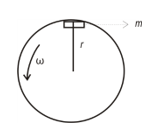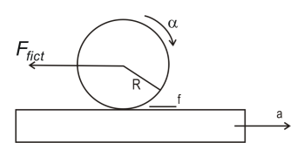# Objective questions on Rotational Motion

In this page we have Important Objective questions on rotation for JEE Main/Advanced,CBSE,AP Physics . Hope you like them and do not forget to like , social share and comment at the end of the page.

## Paragraph Based Questions

(A) Three bodies A(ring) ,B(Solid cylinder) ,C (solid sphere) rolls down the same inclined plane of angle $\alpha$ and height H with out slipping.
The radii of the bodies are identical and equal to R.The mass of the bodies are
M1, M2, M3 respectively. The relation in the masses is given as below
M1 > M2 > M3

Question 1
Statement I: The velocity of the center of mass of the bodies at the bottom of the incline plane is dependent on the mass of the bodies
Statement II: The total kinetic energy( Translation + Rotational) will be largest for the body A at the bottom of the inclined plane
(a) Statement I is true only
(b) Statement II is true only
(c) Both the statements are true
(d) Both of them are false
Question 2
Which one will have largest Center of mass velocity at the bottom of the incline plane
(a) A
(b) B
(c) C
(d) Data insufficient
Question 3
Which one will have least center of mass velocity at the bottom of the incline plane
(a) A
(b) B
(c) C
(d) Data insufficient
Question 4
At any time during the motion, the velocity of the point in contact of the body with the incline plane
(a) 0
(b) 2vcm
(c) vcm
(d) None of the above
Question 5
At any time during the motion, the velocity of the uppermost point of the body
(a) 0
(b) 2vcm
(c) vcm
(d) None of the above
Question 6
The angular velocity will be maximum at the bottom of the incline place for the body
(a) A
(b) B
(c) C
(d) Data insufficient

(B)A nearly mass less rod (AB) of Length L is pivoted at one end A so that it can swing free as a pendulum. Three masses A(m) ,B(2m) and C( 3m) are attached to it at the distance L/4,L/3 and L/2 respectively from end A. The rod is held horizontal and then it is released

Question 7
Find the Moment of the Inertia of system about the pivoted end
(a) $I=\frac{mL^2}{144}$
(b) $I=\frac{14mL^2}{144}$
(c) $I=\frac{151mL^2}{144}$
(d) $I=\frac{149mL^2}{144}$

Question 8
Find the angular acceleration of the rod at the instant it is release
(a) $\alpha=\frac{348g}{14L}$
(b) $\alpha=\frac{348g}{19L}$
(c) $\alpha=\frac{34g}{149L}$
(d) $\alpha=\frac{348g}{149L}$

(C) A Projectile of mass m is fired from point O(origin) with an initial velocity u at angle θ above the horizontal

Question 9
Find the x, y and z component of the angular momentum of the projectile about point O as a function of time
(a) 0,0,$\frac{-m(ucos{\theta})gt^2}{2}$
(b) 0,0,$\frac{m(ucos{\theta})gt^2}{2}$
(c) 0,$u cos \theta$,$\frac{-m(ucos{\theta})gt^2}{2}$
(d) 0,$u cos \theta$,$\frac{m(ucos{\theta})gt^2}{2}$

Question 10
Calculate the torque of the weight acting on the projectile about the Origin
(a)$mgu (cos \theta) t\mathbf{k}$
(b) $mgu (cos \theta) t\mathbf{j}$
(c) $-mgu (cos \theta) t\mathbf{k}$
(d) None of the above

(D) A horizontal turn table carries a gun at point X and rotates with initial angular velocity ω0  about its vertical geometric axis. The Gun fires a bullet of mass m with tangential muzzle velocity vI0 is the moment of inertia of the turntable and gun excluding bullet

Question 11
Find the initial angular momentum of the system about the axis of rotation
(a) $I_0\omega+mr^2\omega$
(b) $I_0\omega$
(c) $I_0\omega-mr^2\omega$
(d) None of these

Question 12
Find the final angular velocity of the turntable
(a)$\omega_0+\frac{mvr}{I_0}$
(b) $\omega_0$
(c) $\omega_0-\frac{mvr}{I_0+mr^2}$
(d) $\omega_0+\frac{mvr}{I_0+mr^2}$

(E)A uniform rod (AB) of length L and mass 2M rest on smooth horizontal surface. A point mass M moving horizontally at right angles to the rod with initial velocity v collides with one end (A) and sticks to it

Question 13
Find the velocity of the center of mass of the system after the collision
(a) v/3
(b) v/2
(c) v/4
(d) 3v/4
Question 14
Find the angular velocity of the rod after the collision
(a)v/L
(b) v/2L
(c) 2v/L
(d) 3v/4L
Question 15
Match the column
Column A -> Velocity of the various part of the rod
Column B -> Their values in terms of v
Column A
(P) Velocity of the end A
(Q) Velocity of the end B
(R) Velocity of the point C which is at distance 2L/3 from point A
(S) Velocity of point D which is at distance L/2 from A
Column B
(U) -v/3
(V) 2v/3
(W) 0
(X) v/6
Question 16
which of the following will remain true in collision
(a) Law of conservation of linear momentum
(b) Law of conservation of angular momentum
(c) Law of conservation of energy
(d) None of these
Question 17
Find the total energy of the system after the collision
(a) $\frac{mv^2}{3}$
(b) $\frac{mv^2}{2}$
(c) $\frac{mv^2}{4}$
(d) $\frac{mv^2}{6}$
Question 18
Which of the following is true?
(a) The Center of mass velocity before and after the collision will remain same
(b) Moment of inertia about center of mass of the rod is
$\frac{2mL^2}{9}$ (c) Net torque and net force is zero on the system
(d) None of these

(F)A rod of length L and mass M can freely rotate around a pivot at A. A bullet of mass m and velocity v hit the rod at a height h from A and becomes imbedded in it

Question 19
Find the angular velocity of the Rod just after the collision
(a) $\frac{mvh}{mh^2+ML^2}$
(b) $\frac{mvh}{mh^2+\frac{1}{3}ML^2}$
(c) $\frac{mvh}{mh^2+\frac{1}{2}ML^2}$
(d) None of these

Question 20
Find the Linear Momentum of the system just after the collision if ω is the angular velocity just after the collision
(a)(mh+ML/2) ω
(b) (mh+ML) ω
(c) (mh+ML/3) ω
(d) None of these

(G)An upright hoop is projected on a pavement with a initial horizontal speed v0 but with out spin so that its slide. The resulting frictional force causes the hoop to spin to loose translational speed and to acquire an angular speed. Eventually the hoop rolls without slipping and let μ be the coefficient of friction
Question 21
Find the time taken by the hoop to start rolling with out slipping
(a) v0/2 μg
(b) v0/2 g
(c) v0/ μg
(d) None of these

Question 22
Find the velocity of the hoop at no slipping
(a) v0/2
(b) 3 v0/2
(c) v0/4
(d) None of these

Question 23
which one of the following statement are correct
(a) The loop will have total energy $\frac{Mv_0^2}{4}$ when it start pure rolling
(b) $\frac{3Mv_0^2}{4}$ Energy is lost in friction during the whole motion
(c) The velocity of the top most point is v0 when it start pure rolling
(d) $\frac{Mv_0^2}{8}$ is the rotational energy of the loop when it start pure rolling

(H)A cylinder of Mass M and radius R is kept on the plank. The plank starts moving with acceleration a. The cylinder rolls without slipping on the plankQuestion 24
Find the acceleration of the cylinder center of mass from the laboratory frame of reference
(a)$\frac{2}{3}a$
(b)$\frac{1}{3}a$
(c)$\frac{1}{2}a$
(d)$\frac{1}{4}a$

Question 25
Find the frictional force acting on the cylinder
(a)$\frac{Ma}{3}$
(b)$\frac{Ma}{2}$
(c)$\frac{2Ma}{3}$
(d) $\frac{Ma}{4}$

Question 26
Find the angular acceleration of the cylinder about the axis passing through center of mass
(a) $\frac{a}{3R}$
(b) $\frac{a}{4R}$
(c) $\frac{a}{2R}$
(d) $\frac{2a}{3R}$

## Multiple Choice Questions

Question 27
Position vector of a body of mass 6 Kg is given as
$\mathbf{r}=\mathbf{i}(3t^2-6t)+\mathbf{j}(-4t^3)$
Find the torque acting on it about the origin
(a) $\mathbf{k}(-288t^3+864t^2)$
(b)$\mathbf{i}(-288t^3+864t^2)$
(c) $\mathbf{j}(-288t^3+864t^2)$
(d) None of these

Question 28
A nearly massless rod is pivoted at one end so it can freely swing as a pendulum. Two masses 2m and m are attached to it at distance a and 3a respectively from the pivot end. The rod is held horizontal and then released.
Which of the following is correct?
(a) The angular acceleration at the instant it is released $\frac{5g}{11a}$
(b) Moment of inertia of the system about pivot is 11ma2
(c) The angular acceleration at the instant when the rod makes an angle θ with horizontal $\frac{5gcos{\theta}}{11a}$
(d) The net torque about the pivot end becomes zero when rod becomes vertical.